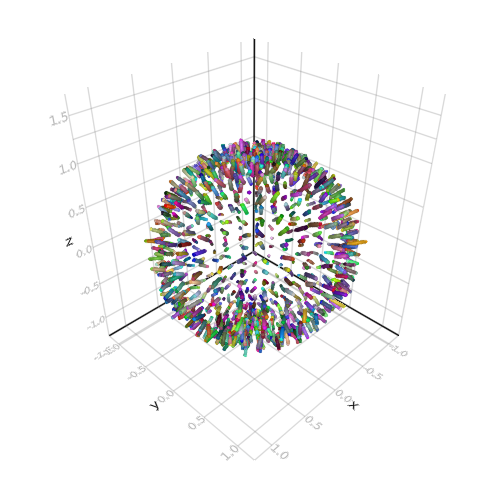## Fluctuation 3D

```
using GeometryBasics, Colors
using GeometryBasics: Cylinder, rotation

using AbstractPlotting

# define points/edges
perturbfactor = 4e1
N = 3; nbfacese = 30; radius = 0.02

large_sphere = Sphere(Point3f0(0), 1f0)
positions = decompose(Point3f0, large_sphere, 30)
np = length(positions)
pts = [positions[k][l] for k = 1:length(positions), l = 1:3]
pts = vcat(pts, 1.1 .* pts + randn(size(pts)) / perturbfactor) # light position influence ?
edges = hcat(collect(1:np), collect(1:np) .+ np)
ne = size(edges, 1); np = size(pts, 1)
cylinder = Cylinder(Point3f0(0), Point3f0(0, 0, 1.0), 1f0)
# define markers meshes
meshC = normal_mesh(cylinder; nvertices=nbfacese)
meshS = normal_mesh(large_sphere; nvertices=20)
# define colors, markersizes and rotations
pG = [Point3f0(pts[k, 1], pts[k, 2], pts[k, 3]) for k = 1:np]
lengthsC = sqrt.(sum((pts[edges[:,1], :] .- pts[edges[:, 2], :]) .^ 2, dims = 2))
sizesC = [Vec3f0(1) for i = 1:ne]
colorsp = [RGBA{Float32}(rand(), rand(), rand(), 1.0) for i = 1:np]
colorsC = [(colorsp[edges[i, 1]] .+ colorsp[edges[i, 2]]) / 2.0 for i = 1:ne]
Qlist = zeros(ne, 4)
for k = 1:ne
ct = Cylinder(
Point3f0(pts[edges[k, 1], 1], pts[edges[k, 1], 2], pts[edges[k, 1], 3]),
Point3f0(pts[edges[k, 2], 1], pts[edges[k, 2], 2], pts[edges[k, 2], 3]),
1f0
)
Q = rotation(ct)
r = 0.5 * sqrt(1 .+ Q[1, 1] .+ Q[2, 2] .+ Q[3, 3]); Qlist[k, 4] = r
Qlist[k, 1] = (Q[3, 2] .- Q[2, 3]) / (4 .* r)
Qlist[k, 2] = (Q[1, 3] .- Q[3, 1]) / (4 .* r)
Qlist[k, 3] = (Q[2, 1] .- Q[1, 2]) / (4 .* r)
end

rotationsC = [Vec4f0(Qlist[i, 1], Qlist[i, 2], Qlist[i, 3], Qlist[i, 4]) for i = 1:ne]
# plot
scene = meshscatter(
pG[edges[:, 1]],
color = colorsC, marker = meshC,
markersize = sizesC,  rotations = rotationsC,
)
meshscatter!(
scene, pG,
color = colorsp, marker = meshS, markersize = radius,
)
scene

```